research papersJOURNAL OFSYNCHROTRONRADIATION
ISSN: 1600-5775
Volume 20| Part 1| January 2013| Pages 145-152

A preliminary design of a knot undulator

aDepartment of Physics, Laboratory of Advanced Materials and Surface Physics Laboratory (National Key Laboratory), Fudan University, No. 220 Handan Rd, Shanghai 200433, People's Republic of China, and bAdvanced Light Source, Lawrence Berkeley National Laboratory, 1 Cyclotron Road, Berkeley, CA 94720, USA
*Correspondence e-mail: qiaoshan@fudan.edu.cn

(Received 4 July 2012; accepted 14 September 2012; online 10 November 2012)

The magnetic field configuration of the previously proposed knot undulator [Qiao et al. (2009). Rev. Sci. Instrum. 80, 085108] is realised in the design of a hybridized elliptically polarized undulator, which is presented. Although the details of the field distribution are not the same as those in the theoretical proposal, it is demonstrated that the practical knot undulator could work perfectly. In order to understand the minor discrepancies of the two, mathematical formulae of the synchrotron radiation are derived based on the Fourier transform of the magnetic field. From the results of calculations by simulation program, the discrepancies could be well interpreted by the corresponding formulae. The results show the importance of optimization of the end sections of the knot undulator to suppress the on-axis heat load. Furthermore, a study of the impact of the undulator on beam dynamics of the storage ring was conducted using the Shanghai Synchrotron Radiation Facility as an example and the results show that the knot undulator has little effect on the beam.

1. Introduction

The heat load on beamline optics is a serious problem for the third-generation synchrotron radiation sources, especially for an ordinary planar undulator that generates linearly polarized light. There are several approaches to obtain linearly polarized radiation with low on-axis heat load, such as crossed elliptically polarized undulators (EPUs) (Kim, 1984), figure-8 (Tanaka & Kitamura, 1995, 2000; Tanaka et al., 2002) and PERA (Sasaki et al., 1998b) undulators. All of them are ingenious in removing the on-axis heat load by the helical movement of the electrons such that the electron velocity is never parallel to the undulator axis. Meanwhile, to take advantage of synchrotron radiation, beamlines which can supply radiation with variable polarization, circular or linear, are highly in demand. An APPLE (Advanced Planar Polarized Light Emitter) (Sasaki et al., 1993) type undulator can satisfy this requirement, but, because it generates linear polarized light by normal linear movement of electrons, the high-heat-load problem cannot be overcome and a final solution is the APPLE-8 (Sasaki et al., 1998a) design. To generate low-energy photons in a high-energy storage ring, a long undulator period is essential and electromagnetic undulators have to be used. However, there had been no good solution to the heat-load problem for electromagnetic undulators until the invention of knot (Qiao et al., 2009) and leaf (Yan & Qiao, 2010) operating modes of electromagnetic EPUs. Knot mode can supply photons with circular or linear polarization (in the vertical or the horizontal direction) with less than 1% of the on-axis heat load generated by normal linearly polarized undulators. On the other hand, leaf mode can supply linear polarized photons in an arbitrary direction of polarization with an on-axis heat load comparable with that of the knot undulator. Both modes operate based on the principle of crossed EPUs where the electrons move alternately in a right-handed and then a left-handed circle which results in the cancellation of circular polarization of photons and leaves as a remnant a pure linear polarization. In a conventional crossed EPU, the decrease of the degree of linear polarization is proportional to the square of the undulator period number (Sasaki et al., 1997) owing to the decrease of the interference between the two right-handed and left-handed polarized EPUs. Knot and leaf undulators can be regarded as a cascaded array of a single-period crossed EPUs to obtain purely linear or circular polarized photons by the adjustment of the phase shift of EPUs. The switch between different modes can be performed simply by the change of the energization pattern of the undulator.

Up to now, knot and leaf operational modes have only been proposed theoretically, i.e. only the ideal distribution of the magnetic field is given theoretically without consideration of the properties of the physical magnets. In the theoretical model the magnetic field By in the Y direction is a normal sinusoidal function, whereas the field Bx in the X direction vanishes in certain segments along the z axis to obtain the necessary phase shift. In practice, the exact zero intensity segments cannot be realised because of the continuity of the derivative of the magnetic field and the cross-talk from adjacent magnets. Although its effect on photon intensity and polarization should be very small from the theoretical point of view that the photons with linear polarization in the X direction are mainly related to By, the characteristics of photons from the real magnetic field need to be studied to verify our consideration. This paper will show a preliminary design of such a knot undulator and compare its characteristics with the idealized theoretical model. Our results demonstrate that the practical knot undulator could work almost the same as the theoretical prediction.

2. Principle and structure

For a knot undulator, the magnetic field By in the Y direction is a normal sinusoidal function with period λu, and the magnetic field Bx in the X direction is a periodic function with period 1.5λu. After Fourier expanding of Bx and By, and ignoring the high-order items (the rationality will be shown later), the magnetic field of the knot undulator can be expressed approximately as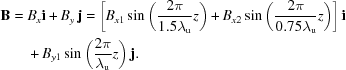Hence the undulator strength of every component can be expressed as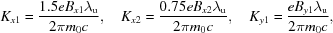where m0 is the mass of electrons.

Inside the undulator, electrons receive a Lorentz force, F =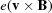. Solving the kinetic equation with the initial conditions, the velocity of electrons can be obtained,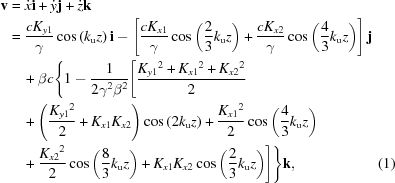where ku =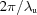is the wavenumber of By.

Thus the average electron velocity along the Z direction is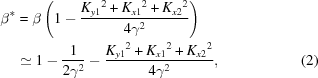where the terms higher than the second order are omitted. The accelerations along the Y and X directions are proportional to the magnetic fields along the X and Y directions, soand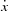are proportional to the integrations of Bx and By, respectively, and oscillate between positive and negative maximums. Because the Lorentz force does no work on electrons, the total velocity remains unchanged and the average velocity along the Z axis decreases with the increase of the magnetic fields along the X and Y axes as shown in equation (2).

The position of an electron in the undulator can be derived from the integrations of velocities,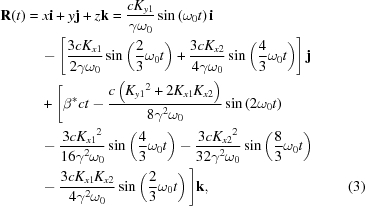where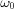=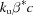.

The above equations are derived based on the laboratory frame. Now we consider a coordinate system moving along the Z axis with a velocity equal to the averagein the laboratory frame, that is,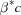. The movement of electrons in this frame is just a composition of harmonic oscillations in the X (with a frequency of ω0), Y [with two frequencies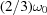and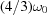] and Z directions. The dipole harmonic oscillation generates polarized monochromatic light with the same frequency and the polarization is in the plane determined by directions of oscillation and observation, and perpendicular to the observation direction; and no light will be generated if the propagating direction of light is along the oscillation direction. In our case, if we observe the light around the Z axis, the electrons oscillated along the Z direction generate no light and we can observe X polarized light with frequency ω0 and Y polarized light with two frequenciesand. After taking into account the relativistic Doppler shift, the frequency of photons ω1 observed in laboratory coordinates should be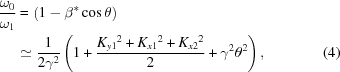when θ, which is the angle between the axis of the undulator and the observation direction, is small.

The brightness of the undulator can be derived (Duke, 2000) as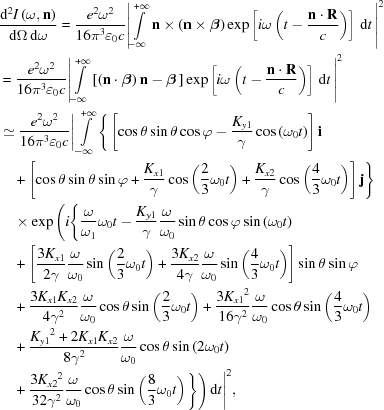whereis the unit vector along the observation direction.

In the spherical coordinate frame,=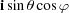+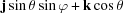, the brightness can be simplified as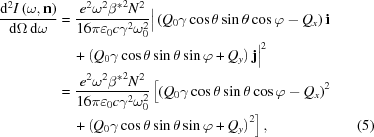where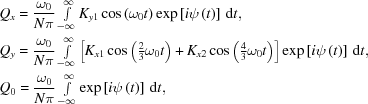where the phase is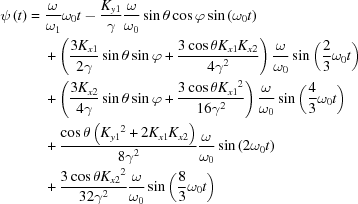and Qx, Qy, Q0 are functions of ω, θ and φ.

Although equation (5)is complicated, in particular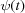is relative to both Bx and By, it still can show some information intuitively. The term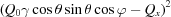is proportional to the photon intensity polarized in the X direction, and there is a positive correlation between By12 and the X polarized photon intensity. Also, the photon intensity polarized in the Y direction is determined by the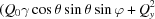term and Qy is mainly determined by Bx.

3. Design of a knot undulator

As reported by Qiao et al. (2009), a theoretical model of a knot undulator to supply photon energy ranging from 7 to 70 eV was proposed for the Shanghai Synchrotron Radiation Facility (SSRF; 3.5 GeV). A more realistic configuration, based on the superposition of a permanent-magnet Hallbach arrangement with a superimposed electromagnet configuration, has been developed to determine the general feasibility of the concept (see Fig. 1). The vertical and horizontal magnetic fields are generated by permanent and electric magnets, respectively, because the shift from one operation mode (for example, normal EPU) to the other (for example, knot) can be achieved with the unchanged vertical field. Owing to the horizontal space constraints of the vacuum chamber and the various modes of operation envisioned for such a device, the magnet configurations do not incorporate magnetic materials (e.g. magnetic steels), but rely on pure permanent-magnet and electromagnet coils only. Note that this approach is limited by the current density that can be carried by the coils; whereas some attempt was made to optimize the geometry of the coils, no detailed thermal analysis has been performed to determine the limiting field value that can be obtained from the coils. A detailed design study would also be required to determine the permeance (i.e. load-line) in the permanent-magnet material under various operating conditions, so as to avoid demagnetization of the material.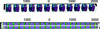Figure 1 Magnetic coils (upper) and permanent-magnet array (bottom) of an undulator that produce the desired horizontal and vertical fields, respectively. The permanent-magnet array is sandwiched between the coils.

One advantage of not having permeable steels in the magnetic system is that reasonably fast field variations can be envisioned without significant hysteresis or eddy-current effects. Eddy currents in the vacuum chamber are typically negligible, unless very fast field changes are needed. The small relative permeability of the permanent-magnet material is not expected to result in hysteresis, based on operating experience with APPLE-II devices on a large number of light sources worldwide.

The magnetic field of the knot undulator design (solid line in Fig. 2) is obtained based on the electromagnet arrangement calculated with the static magnetic field computation code RADIA (Elleaume et al., 1997). The damped permanent magnetic field at the ends is designed to minimize first and second field integrals at all gaps, although no effort was made here to generate optimal end configurations.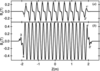Figure 2 Magnetic fields of the practical (solid line) and theoretical (dashed line) knot undulator. (a) Horizontal magnetic field Bx with an amplitude of 0.252 T for the practical and 0.29 T for the theoretical undulator. (b) Vertical magnetic field By with an amplitude of 0.5 T for both models.

Fig. 2shows the magnetic fields of the theoretical and the practical undulators. While the magnetic fields in the Y direction are almost the same, significant difference occurs in the regions where Bx vanishes in the theoretical design. Instead of being zero over a finite length, Bx of the practical one changes gradually from positive to negative owing to the influence of neighbour magnets as we expected. To ensure that we can obtain the identical fundamental photon energy, the average velocities of electrons in both cases have to be the same. Because the characteristics of the fundamental radiation with linear polarization along the X direction is mainly determined by By, the amplitudes of By are selected to be the same and the amplitude of Bx p is adjusted to keep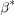in the practical undulator equal to that in the theoretical one. To keep the axis of the electron orbit coinciding with the axis of the undulator, some end magnetic field sections (noted by 1, 2, 3 and 4 in Fig. 2) are inevitable. To exclude their effects when comparing the performance, similar end magnetic fields are adopted for both the theoretical and the practical designs. The end fields in regions 1 and 2 are slightly smaller and the end field in region 3 is slightly larger for the theoretical one than those of the practical one in order to ensure coincidence of the two axes.

4. Effect of the undulator on beam dynamics

To verify the feasibility of the device, we studied its effect on the single particle dynamics using the storage ring at the SSRF as an example. The undulator is placed at the centre of one of the long straight sections with no further adjustment of the machine. Frequency map analysis (Laskar, 1990, 1993; Laskar et al., 1992; Dumas & Laskar, 1993; Robin et al., 2000) of the ring with the undulator on and off was performed using the code Accelerator Toolbox (Terebilo, 2001). The relative random errors of the quadrupole strengths and the gradients of the bending magnets are 0.1% r.m.s. and those of the skew quadrupole components are 0.3% r.m.s., which are typical for a third-generation light source. The results are presented in Figs. 3–6, which show that the impact of the undulator on the lattice functions and the dynamic aperture is rather small. Furthermore, adjacent quadrupoles and/or a quadrupole corrector on the undulator can be used to cancel the residual focusing of the undulator, thus removing the β and dispersion beating and making the undulator virtually transparent.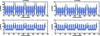Figure 3 β-functions of the SSRF storage ring with the undulator off (left) and on (right).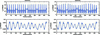Figure 4 Dispersion functions of the SSRF storage ring with the undulator off (left) and on (right).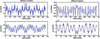Figure 5 Differences in β and dispersion functions of the SSRF storage ring with the undulator off and on. The tunes and chromaticities are adjusted to the same values and no other errors are included. The absence of vertical dispersion generated by the undulator shows that the undulator does not generate coupling between the horizontal and the vertical planes. In principle, the β-beating generated by the undulator can be largely corrected using the adjacent quadrupoles. The figures at the bottom show that this level of disturbance does not affect the beam dynamics significantly.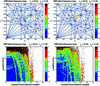Figure 6 Tunes (top) and transverse positions (bottom) of the SSRF storage ring with the undulator off (left) and on (right). The colour denotes the diffusion rate of the electrons, which is defined as the difference of tunes per turn of the first and second half of the turns.

5. Results and discussions

The Fourier expansions of the theoretical and the practical magnetic fields in the X and Y directions are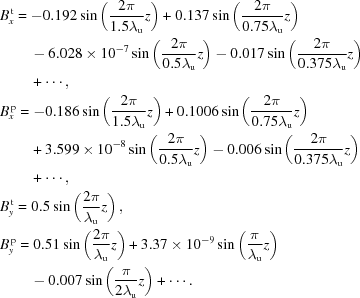The terms higher than the second order are small in both Bx t and Bx p, and it is enough to retain the preceding two terms in the following calculations. The magnetic field in the Y direction of the practical design is not a perfect sinusoid, but we can see that the high-order terms are very small compared with the first-order term and can be omitted in the following calculations. So we can use the formulae derived previously.

As a result, we can obtain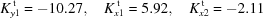for the theoretical one and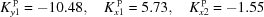for the practical one. Furthermore, we obtain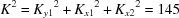for both designs to ensure the same fundamental photon energy.

The flux of photons in the 0.6 mrad × 0.6 mrad acceptance solid angle is equal to the integral of equation (5), which is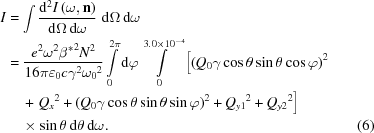The flux ratio of the practical and the theoretical designs I p/I t can be estimated from the above equation through numerical calculation and the result is 1.01 when the photon energy is 7 eV. To verify the correctness of (6), the photon flux in the energy range from 4.0 to 14.6 eV is calculated based on equation (6)[dots in the inset of Fig. 9(a)] and the software SPECTRA (version 8.1) under the condition of zero energy spread [solid line in the inset of Fig. 9(a)]. Obviously they match well and the error is 0.3% at 7 eV.

Also we can calculate the detailed performance of the practical and the theoretical designs with well developed software. The following calculations were performed using the software SPECTRA (version 8.1), developed by Tanaka & Kitamura (2001), with the storage ring parameters of SSRF. The trajectory of the electrons and the relative velocities are shown in Fig. 7. The projection of the electrons' trajectory on the XY plane shows successive right- and then left-handed movements and forms a complex knot figure as shown in Fig. 7(a), and the largest discrepancy between the practical (solid line) and the theoretical (dashed line) designs are a few micrometres. It is well predicted by equation (3). It should be noted that the orbit of electrons at the entrance and exit of the knot undulator changes gradually owing to the end field sections, and the electrons do not move in the stable knot figure orbit immediately, as shown in Fig. 7. There are some discrepancies between the velocities of electrons in the practical and the theoretical models as shown in Fig. 7(b). First, the AB and FG sections in the practical model form a feeler-like curve corresponding to the gradually changed magnetic field from positive to negative, whereas the same sections in the theoretical model form a straight line, which is a result of the zero magnetic field in this section. Secondly, section HIJK forms a quasi-elliptical curve for the practical model but is exactly elliptical for the theoretical model. The deviation from an elliptical figure is due to the fact that Bx of the practical model is not exactly sinusoidal as shown in Fig. 2(a). The discrepancies of velocities between theoretical and practical models are predicted well by equation (1). For the practical model, the velocity of electrons is also never parallel to the undulator axis as in the case of the theoretical model. Hence it can guarantee a low on-axis heat load as we expected.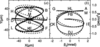Figure 7 (a) Trajectory of the electrons projected onto the XY plane. (b) Relative velocities βy versus βx of the electrons; the solid and dashed lines are for the practical and theoretical knot models, respectively.

The spatial distribution of photon intensity for 7 eV photons is shown in Figs. 8(b) and 8(d). Almost all of the photons can be collected for both the practical and the theoretical models if we select an acceptance angle of 0.6 mrad in both X and Y directions. The spatial distributions of total power density, including fundamental and harmonic photons, are shown in Figs. 8(a) and 8(c)for the practical and the theoretical models, respectively. Their shapes are similar to the velocity distribution shown in Fig. 7(b)and so most power is far from the undulator axis and can be removed easily by the aperture. The total power is 895.5 W and 894.7 W for the practical and the theoretical models, respectively. The power of photons in the 0.6 mrad × 0.6 mrad acceptance solid angle from the practical and the theoretical undulators is 7.31 W and 6.40 W, respectively. It is larger than 1.93 W which is the on-axis heat load produced by electrons in a stable knot orbital (Qiao et al., 2009). Although the electrons only pass a short distance to enter the stable knot orbital, calculation results show that the most part of the on-axis heat load is from electrons in the entrance and exit sections of the knot undulator, because the magnetic field in these sections is weak (see Fig. 2), resulting in the small velocities in the xand y directions. So, in order to obtain a lower on-axis heat load, it is important to optimize the end field sections to force the electrons to move in a path deviated from the undulator axis and enter the stable knot orbital more quickly.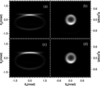Figure 8 Spatial distribution of (a) power density and (b) photon intensity of 7 eV fundamental harmonics for the practical undulator. (c, d) Corresponding distributions for the theoretical undulator.

Fig. 9shows the photon flux and corresponding linear polarization inside a 0.6 mrad × 0.6 mrad acceptance solid angle from the practical (solid line) and the theoretical (dashed line) undulators. We can see that both the flux and polarization of the practical and the theoretical models are almost the same for 7 eV photons. Although the amplitude of the field in the Y direction of the practical model is 0.5 T, the same as that of the theoretical model, the amplitude of its first-order Fourier component is slightly larger, 0.51 T, owing to the fact that it deviates from sinusoidal slightly. This results in a stronger photon intensity [1.65 × 1014 photons s−1 (0.1% bandwidth)−1 (100 mA)−1] at 7 eV compared with the theoretical model [1.57 × 1014 photons s−1 (0.1% bandwidth)−1 (100 mA)−1], a 5% increase, which agrees well with our estimation. The linear polarizations at 7 eV for the practical and the theoretical models are 98.2% and 98.3%, respectively.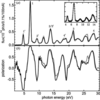Figure 9 (a) Comparison of photon fluxes from the theoretical (dashed line) and practical (solid line) undulators. The acceptance angles are 0.6 mrad in both the vertical and horizontal directions. The inset shows the photon fluxes calculated using equation (6)(dots) and SPECTRA under the condition of zero energy spread (solid line). (b) The corresponding linear polarization curves. Plus and minus signs of the polarization axis correspond to polarizations in the X and Y directions.

As pointed out by Qiao et al. (2009), the spectrum of the knot undulator has two series of peaks. The peaks noted by 1, 2, 3 and 4 in Fig. 9(a)correspond to the harmonics related to the field in the Y direction whose period is 220 mm and their polarization is horizontal. For all of them, the intensities of the practical model are stronger than those of the theoretical one owing to the larger magnetic field By. The peaks noted by 1′, 2′, 3′ and 4′ are the harmonics of the 330 mm-period magnetic field in the X direction and their polarization is vertical. Their intensities in the practical model are weaker than those in the theoretical model owing to the smaller Bx.

6. Conclusions

The practical design of the knot undulator can work just as well as the idealized theoretical model with low on-axis heat load, strong fundamental photon intensity and high linear polarization. To explain the minor discrepancies between the two models, we derived the formulas to calculate the velocity, trajectory and photon intensity based on the Fourier expansion of the magnetic fields which could interpret well the discrepancies between the practical and theoretical knot undulators. The impact of the undulator on beam dynamics is negligible. Our results also show the importance of the optimization of the end sections of knot undulators to achieve a low on-axis heat load.

Acknowledgements

This work is supported by the Natural Science Foundation of China (No. 10979021, 11027401 and 11174054), the Ministry of Science and Technology of China (National Basic Research Program No. 2011CB921800) and the Shanghai Municipal Education Commission. One of the authors (WW) thanks Dr Manzhou Zhang for providing the lattice file of the SSRF storage ring.

ReferencesJOURNAL OFSYNCHROTRONRADIATION